Home

# Two-dimensional cross-spectrum of the streamwise velocity in turbulent boundary layers

## Abstract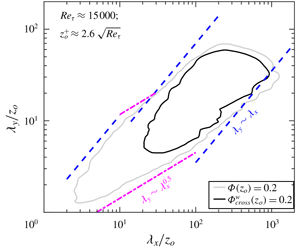In this paper, we present the two-dimensional (2-D) energy cross-spectrum of the streamwise velocity (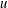$u$ ) component and use it to test the notion of self-similarity in turbulent boundary layers. The primary focus is on the cross-spectrum (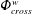$\unicode[STIX]{x1D6F7}_{cross}^{w}$ ) measured across the logarithmic (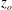$z_{o}$ ) and near-wall (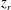$z_{r}$ ) wall-normal locations, providing the energy distribution across the range of streamwise (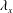$\unicode[STIX]{x1D706}_{x}$ ) and spanwise (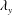$\unicode[STIX]{x1D706}_{y}$ ) wavelengths (or length scales) that are coherent across the wall-normal distance.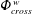$\unicode[STIX]{x1D6F7}_{cross}^{w}$ may thus be interpreted as a wall-filtered subset of the full 2-D$u$ -spectrum (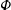$\unicode[STIX]{x1D6F7}$ ), the latter providing information on all coexisting eddies at$z_{o}$ . To this end, datasets comprising synchronized two-point$u$ -signals at$z_{o}$ and$z_{r}$ , across the friction Reynolds number range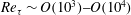$Re_{\unicode[STIX]{x1D70F}}\sim O(10^{3}){-}O(10^{4})$ , are analysed. The published direct numerical simulation (DNS) dataset of Sillero et al. (Phys. Fluids, vol. 26 (10), 2014, 105109) is considered for low-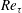$Re_{\unicode[STIX]{x1D70F}}$ analysis, while the high-$Re_{\unicode[STIX]{x1D70F}}$ dataset is obtained by conducting synchronous multipoint hot-wire measurements. High-$Re_{\unicode[STIX]{x1D70F}}$ cross-spectra reveal that the wall-attached large scales follow a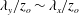$\unicode[STIX]{x1D706}_{y}/z_{o}\sim \unicode[STIX]{x1D706}_{x}/z_{o}$ relationship more closely than seen for$\unicode[STIX]{x1D6F7}$ , where this self-similar trend is obscured by coexisting scales. The present analysis reaffirms that a self-similar structure, conforming to Townsend’s attached eddy hypothesis, is ingrained in the flow.

## References

Hide All
MathJax
MathJax is a JavaScript display engine for mathematics. For more information see http://www.mathjax.org.

# Two-dimensional cross-spectrum of the streamwise velocity in turbulent boundary layers

## Metrics

### Full text viewsFull text views reflects the number of PDF downloads, PDFs sent to Google Drive, Dropbox and Kindle and HTML full text views.

Total number of HTML views: 0
Total number of PDF views: 0 *Loading metrics...

### Abstract viewsAbstract views reflect the number of visits to the article landing page.

Total abstract views: 0 *Loading metrics...

* Views captured on Cambridge Core between <date>. This data will be updated every 24 hours.

Usage data cannot currently be displayed.